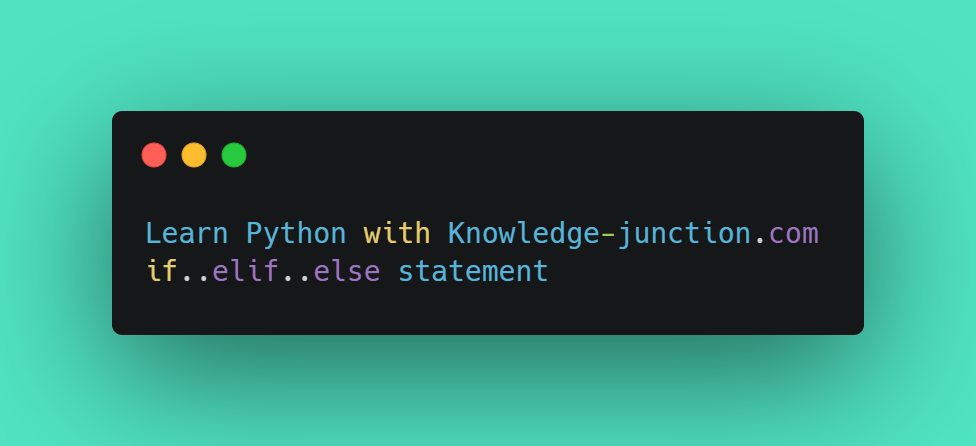# Python : if…else statementHello Friends,

Today in this article we are going to learn if…else statement in Python

What is if…else statement?

We all know that decision is required when we want to execute a code only if a certain condition is satisfied.

The if…elif…else statement is used in Python for decision making.

Syntax of if…else

The `if..else` statement study the `test expression` and will execute the body of `if` only when the test condition is `True`.

If the condition is `False`, the body of `else` is executed.

Python if…else flow chart

Example of if…else

``````num=5

if num >= 0:
print("The number is positive or zero")
else:
print("Number is negative")
----------------------------------------------
In runfile (...)
The number is positive or zero``````

In the above example, when num is equal to 5, the test expression is true and the body of if is executed and the `body` of else is skipped.

We can also try this to numbers

``````#num = 0
#num = -7``````

Python if…elif…else Statement

Syntax of if…elif…else

The `elif` is short for else if. It allows us to check for multiple expressions.

If the condition for `if` is `False`, it checks the condition of the next `elif` block and so on.

If all the conditions are `False`, the body of else is executed.

Only one block among the several `if...elif...else` blocks is executed according to the condition.

Flowchart of if…elif…else

Example of if…elif…else

``````num = 6.5

if num > 0:
print("The number is positive")
elif num == 0:
print("Zero")
else:
print("The number is negative")
------------------------------------------
In runfile (...)
The number is positive``````

When variable num is positive, `The number is positive` is printed.

If num is equal to 0, Zero is printed.

If num is negative, The number is negative is printed.